1.6 Real Numbers:

We have studied properties of  Natural numbers, Whole Numbers, Integers, fractions, irrational number such as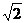and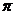. We have also studied that every non zero number has a negative number associated with it such that their sum is zero.

The combined set of rational numbers and irrational numbers is called ‘real number’ and is denoted  by R. Note that a number can be either a rational number or an irrational number and it can not be both.

Therefore

If Q is set of rational numbers and Ir is set of irrational numbers then we say Q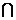Ir =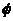(null set)

The relationship between various types of numbers can be represented in a tree structure as follows:

If

N is Set of Natural numbers, W is set of Whole numbers, Z is set of Integers ,Q is set of Rational numbers, R is set of Real numbers and Ir  is set of Irrational numbers

then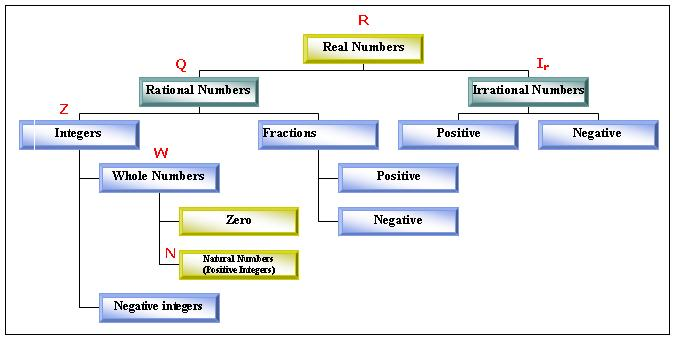The above relationship can also be expressed using a Diagram (called Venn diagram) as follows: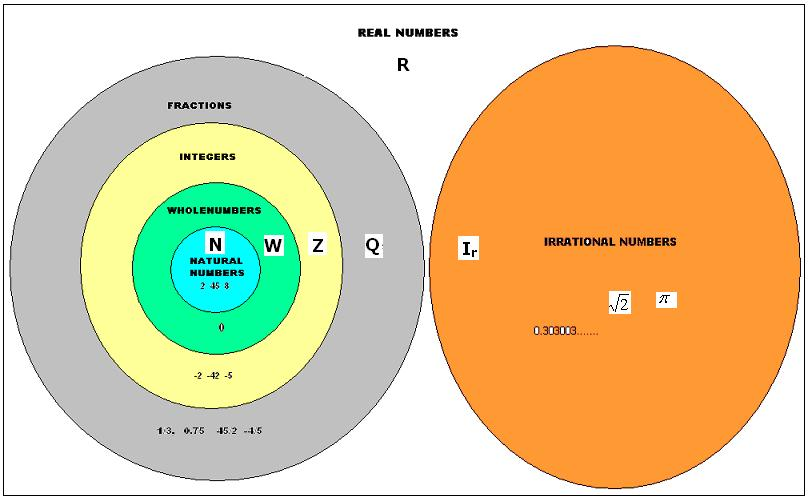Notice  that

N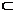WZQR and IrR and Q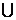Ir = R

If a, b, c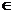R where R is the set of real numbers then

 No Relationships Name of  the property 1 a=a Reflexive property 2 If a=b then b=a Symmetric property 3 If a=b and b=c then a=c Transitive property 4 If a=b then a+c =b+c, ac=bc 5 If ac=bc and c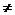0then a=b 6 a+bR Closure property of addition 7 a-bR Closure property of subtraction 8 a*bR Closure property of multiplication 9 a/bR provided b0 Closure property of division 10 a+b = b+a Commutative property of addition 11 a*b = b*a Commutative property of multiplication 12 (a+b)+c = a+(b+c) Associative property of addition 13 a*(b*c) = (a*b)*c Associative property of  multiplication 14 a*(b+c) = a*b + a*c, (b+c)*a = b*a+c*a Distributive law 15 a+0 =0+a =a 0 is additive  identity 16 a*1= 1*a=a 1 is multiplicative  identity 17 a+ (-a) = 0 -a, the additive inverse exists  for every a 18 a*1/a =1 provided a0 1/a,  the multiplicative inverse exists for every a

If a, b and c are real numbers then their order relations are:

 1 Either a=b or ab 2 If a a 3 If a0 Then ac > bc  if c<0

1.6 Problem 1: Solve (x-3)/x2+4 >= 5/x2+4

Solution:

Solve means finding value of x

Multiplying both sides of the given statement by x2+4 we get

(x-3)>= 5(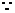x2+4 >0)x >=5+3 (Add 3 to both sides)x >=8

Verification: By substituting value of x =8,9 notice that the the given statement is satisfied.

Euclid's Lemma

Lemma  is a proven statement which is used to prove another statement

We know that

Dividend = (Divisor*Quotient)+Reminder with 0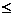reminder< Divisor

This relationship is called Euclid's Division Lemma.

It is also stated as follows:

For any positive  integers a and b there exists unique integers q and r such  that

a=b*q+r  with 0r<b

Alternate way of finding HCF:

We  have learnt in earlier class finding of HCF  by factorisation method and division method.

Using Euclid's division lemma, we can also find HCF.

In this method, starting with  small number as first divisor, we successively divide divisor of each step by reminder of that step till reminder becomes zero, Then the last divisor is HCF.

1.6 Problem 2: Find HCF of 305 and 793

Solution:

 Divisor Division Quotient Reminder 305 305)793(2       610 2 183 183 183)305(1       183 1 122 122 122)183(1        122 1 61 61 61)122(1      122 2 0

Thus 61 is HCF.

1.6 Problem 3:  The length and breadth of a rectangular field is 110m and 30m respectively. Calculate the length of the longest rod which can measure the length and breadth of the field exactly.

Solution:

 Divisor Division Quotient Reminder 30 30)110(3       90 3 20 20 20)30(1      20 1 10 10 10)20(2      20 2 0

Thus HCF is 10

With a rod of length of 10M, we can measure the length and breadth of the field exactly.

A natural number(>1) which is not prime is called composite number

Note that 24= 2*2*2*3= 3*2*2*2 and 55=11*5= 5*11

Thus, every composite number can be expressed as product of primes in a unique way ignoring the order of the terms. This us called fundamental theorem of arithmetic.

1.6 Problem 3:  Find HCF and LCM  of 18,81 and 108

Solution:

18=2*9=  2*32

81=9*9=34

108 = 12*9 = 4*3*9 = 22*33HCF = 32=9LCM = 22*34= 4*81= 324

Note  that HCF*LCM of 3 numbersproduct of 3 numbers

Theorem: If a prime number p divides a2 then p divides a, where  a is natural number > 1

Proof : Let a = p1*p2*p3* … *pn  where   p1,p2,p3, … ,pn  are prime factors of a

Squaring both sides gives

a2=( p1*p2*p3* … *pn)2

Since it is given that p divides a2,  p  is a factor of p12*p22*p32* … *pn2p has to be one among   p1,p2,p3, … ,pn .

Hence the conclusion is that p divides a

1.6 Problem 3:  Prove that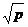where p is a prime number is irrational

Solution:

Assumeis not rational, then= a/b( simplest form)pb2= a2=( p1*p2*p3* … *pn)2 where   p1,p2,p3, … ,pn  are prime factors of a.

This means that p  divides  a( p is a factor of a)  and hence a=kp for some value of kpb2=k2p2b2= k2pp is a factor of b

In the earlier step we have concluded that p is a factor a. now we are concluding that p is a factor of b also.

This means p divides both a and b which is a contradiction as we  have said earlier that a/b  is in simplest form.

Note that

Rational number+ Irrational number = Irrational number( Ex. 3+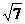)

Rational number*Irrational number = Irrational number( Ex 4)

1.6 Summary of learning

 No Points studied 1 2   3 4 Real numbers and their properties Relationship between real numbers and other types of numbers. Sum of rational and irrational number is irrational Product of rational and irrational number is irrational